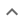•# Simplification or other simple results

We think you wrote:

###This solution deals with simplification or other simple results.

Solution founda*(2a+1)*(2a+5)

## Step by Step Solution## Step  1  :

#### Equation at the end of step  1  :

  ((4 • (a3)) +  (22•3a2)) +  5a
Step  2  :

#### Equation at the end of step  2  :

  (22a3 +  (22•3a2)) +  5a


## Step  4  :

#### Pulling out like terms :

Pull out like factors :

4a3 + 12a2 + 5a  =   a • (4a2 + 12a + 5)

#### Trying to factor by splitting the middle term

Factoring  4a2 + 12a + 5

The first term is,  4a2  its coefficient is  4 .
The middle term is,  +12a  its coefficient is  12 .
The last term, "the constant", is  +5

Step-1 : Multiply the coefficient of the first term by the constant   4 • 5 = 20

Step-2 : Find two factors of  20  whose sum equals the coefficient of the middle term, which is   12 .

 -20 + -1 = -21 -10 + -2 = -12 -5 + -4 = -9 -4 + -5 = -9 -2 + -10 = -12 -1 + -20 = -21 1 + 20 = 21 2 + 10 = 12 That's it

Step-3 : Rewrite the polynomial splitting the middle term using the two factors found in step 2 above,  2  and  10
4a2 + 2a + 10a + 5

Step-4 : Add up the first 2 terms, pulling out like factors :
2a • (2a+1)
Add up the last 2 terms, pulling out common factors :
5 • (2a+1)
Step-5 : Add up the four terms of step 4 :
(2a+5)  •  (2a+1)
Which is the desired factorization

## Final result :

  a • (2a + 1) • (2a + 5)